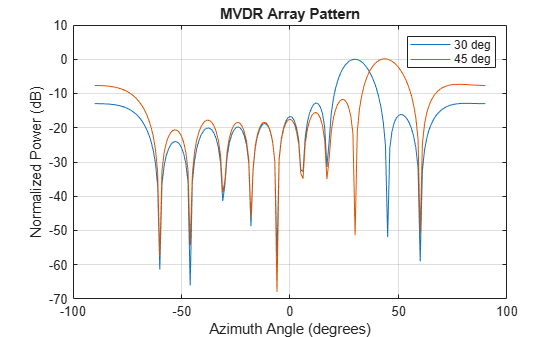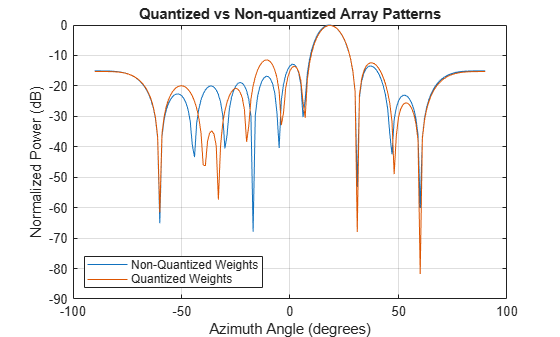Main Content

# mvdrweights

Minimum variance distortionless response (MVDR) beamformer weights

## Syntax

``wt = mvdrweights(pos,ang,cov)``
``wt = mvdrweights(pos,ang,nqbits)``

## Description

example

````wt = mvdrweights(pos,ang,cov)` returns narrowband minimum variance distortionless response (MVDR) beamformer weights for a phased array. When applied to the elements of an array, the weights steer the response of a sensor array in a specific arrival direction or set of directions. The `pos` argument specifies the sensor positions of the array. The `ang` argument specifies the azimuth and elevation angles of the desired response directions. `cov` is the sensor spatial covariance matrix between sensor elements. The output argument, `wt`, is a matrix contains the beamformer weights for each sensor and each direction. Each column of `wt` contains the weights for the corresponding direction specified in `ang`. All elements in the sensor array are assumed to be isotropic.```

example

````wt = mvdrweights(pos,ang,nqbits)` returns quantized narrowband MVDR beamformer weights when the number of phase shifter bits is set to `nqbits`.```

## Examples

collapse all

Construct a 10-element, half-wavelength-spaced line array. Choose two arrival directions of interest - one at 30° azimuth and the other at 45° azimuth. Assume both directions are at 0° elevation. Compute the MVDR beamformer weights for each direction. Specify a sensor spatial covariance matrix that contains signals arriving from -60° and 60° and noise at -10 dB.

Set up the array and sensor spatial covariance matrix.

```N = 10; d = 0.5; elementPos = (0:N-1)*d; Sn = sensorcov(elementPos,[-60 60],db2pow(-10));```

Solve for the MVDR beamformer weights.

`w = mvdrweights(elementPos,[30 45],Sn);`

Plot the two MVDR array patterns.

```plotangl = -90:90; vv = steervec(elementPos,plotangl); plot(plotangl,mag2db(abs(w'*vv))) grid on xlabel('Azimuth Angle (degrees)'); ylabel('Normalized Power (dB)'); legend('30 deg','45 deg'); title('MVDR Array Pattern')```The figure shows plots for each beamformer direction. One plot has the expected maximum gain at 30 degrees and the other at 45 degrees. The nulls at -60 and 60 degrees arise from the fundamental property of the MVDR beamformer of suppressing power in all directions except for the arrival direction.

Construct a 10-element, half-wavelength-spaced line array. Choose the arrival direction of interest to be 18.5° azimuth and 10° elevation. Compute the MVDR beamformer weights and then compute the weights for 3-bit quantization. Specify a sensor spatial covariance matrix that contains signals arriving from -60° and 60° and noise at -10 dB.

Set up the array and the sensor spatial covariance matrix.

```N = 10; d = 0.5; elementPos = (0:N-1)*d; SN = sensorcov(elementPos,[-60 60],db2pow(-10));```

Solve for the MVDR beamformer weights with and without quantization.

```w = mvdrweights(elementPos,[18.5;10],SN); wq = mvdrweights(elementPos,[18.5;10],SN,3);```

Plot both MVDR array patterns.

```plotangl = -90:90; vv = steervec(elementPos,plotangl); plot(plotangl,mag2db(abs(w'*vv))) hold on plot(plotangl,mag2db(abs(wq'*vv))) grid on xlabel('Azimuth Angle (degrees)') ylabel('Normalized Power (dB)') legend('Non-Quantized Weights','Quantized Weights','Location','SouthWest'); title('Quantized vs Non-quantized Array Patterns') hold off```## Input Arguments

collapse all

Positions of the elements of a sensor array specified as a 1-by-N vector, a 2-by-N matrix, or a 3-by-N matrix. In this vector or matrix, N represents the number of elements of the array. Each column of `pos` represents the coordinates of an element. You define sensor position units in term of signal wavelength. If `pos` is a 1-by-N vector, then it represents the y-coordinate of the sensor elements of a line array. The x and z-coordinates are assumed to be zero. When `pos` is a 2-by-N matrix, it represents the (y,z)-coordinates of the sensor elements of a planar array. This array is assumed to lie in the yz-plane. The x-coordinates are assumed to be zero. When `pos` is a 3-by-N matrix, then the array has arbitrary shape.

Example: `[0,0,0; 0.1,0.4,0.3;1,1,1]`

Data Types: `double`

Beamforming directions specified as a 1-by-M vector or a 2-by-M matrix. In this vector or matrix, M represents the number of incoming signals. If `ang` is a 2-by-M matrix, each column specifies the direction in azimuth and elevation of the beamforming direction as `[az;el]`. Angular units are specified in degrees. The azimuth angle must lie between –180° and 180° and the elevation angle must lie between –90° and 90°. The azimuth angle is the angle between the x-axis and the projection of the beamforming direction vector onto the xy plane. The angle is positive when measured from the x-axis toward the y-axis. The elevation angle is the angle between the beamforming direction vector and xy-plane. It is positive when measured towards the positive z axis. If `ang` is a 1-by-M vector, then it represents a set of azimuth angles with the elevation angles assumed to be zero.

Example: `[45;10]`

Data Types: `double`

Sensor spatial covariance matrix specified as an N-by-N, complex-valued matrix. In this matrix, N represents the number of sensor elements.

Example: `[5,0.1;0.1,2]`

Data Types: `double`
Complex Number Support: Yes

Number of bits used to quantize the phase shift in beamformer or steering vector weights, specified as a non-negative integer. A value of zero indicates that no quantization is performed.

Example: `5`

## Output Arguments

collapse all

Beamformer weights returned as a complex-valued, N-by-M matrix. In this matrix, N represents the number of sensor elements of the array while M represents the number of beamforming directions. Each column of `wt` corresponds to a beamforming direction specified in `ang`.

## More About

collapse all

### Minimum Variance Distortionless Response

MVDR beamformer weights minimize the total array output power while setting the gain in the desired response direction to unity (see Van Trees , p. 442). MVDR weights are given by

`$w=\frac{{S}^{-1|}{v}_{0}}{{v}_{0}{}^{H}{S}^{-1}{v}_{0}}$`

where ${v}_{0}$ is the steering vector corresponding to the desired response direction. S is the spatial covariance matrix. The covariance matrix consists of the variances of the element data and the covariances of the data between the sensor elements. The covariance contains contributions from all incoming signals and noise.

 Van Trees, H.L. Optimum Array Processing. New York, NY: Wiley-Interscience, 2002.

 Johnson, Don H. and D. Dudgeon. Array Signal Processing. Englewood Cliffs, NJ: Prentice Hall, 1993.

 Van Veen, B.D. and K. M. Buckley. “Beamforming: A versatile approach to spatial filtering”. IEEE ASSP Magazine, Vol. 5 No. 2 pp. 4–24.

## Support

#### Exploring Hybrid Beamforming Architectures for 5G Systems

Download white paper